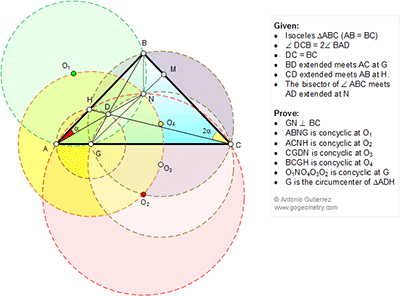## Saturday, November 7, 2015

### Geometry Problem 1163: Isosceles Triangle, Congruence, Double Angle, Circle, Concyclic Points, Circumcenter, Perpendicular, Angle Bisector

Geometry Problem. Post your solution in the comments box below.
Level: Mathematics Education, High School, Honors Geometry, College.

This entry contributed by Sumith Peiris, Moratuwa, Sri Lanka.
Click the diagram below for more details.#### 3 comments:

1.(i) C is the image of A in line BN.

So <BCN = alpha = <DCN.

BN bisects <ABC.

Triangle CBD is isosceles with CB = CD

So CN perp BD (BG).

Together with BN perp GC, we conclude

N is the ortho center of ΔBGC.

Hence GN perp BC

(ii) <BGN =< between GB, GM

= < between their perps CB, CN = alpha =<BAN.

So A,B, N, G are concyclic (O1).

(iii) From (i) <HCN = alpha = <HAN.

So A, C, N, H are concyclic (O2)

(iv) From (ii) <DGN(BGN) = <BAN = alpha

Also <DCN = alpha since CN bisects <BCD

So C, G, D, N are concyclic (O3)

(To be Contd ...)

2.That's only a hint, solution and requirement being long! Key is to prove <ABC=120-2\alpha, which comes easily from: If P is projection of B on AD, from given angular relation we get BD=2 BP, or <BDP=30, thence <DAC=30, so GD=AG ( 1 ) next <BHC=<BGC=60, etc.... CBHG cyclic means tr HGD ~ tr DCB, but DCB is isosceles, so GD=GH and, with (1) last requirement is done.
For the concyclic centers I think the problem would have been extremely tough if not given G and N, because it is very easy to prove each center is at GN distance from G!
Best regards.

3.http://s3.postimg.org/eer166gj7/pro_1163.png

Locations of point D and G :
Locate a point S so that tri. SBC is equilateral.
Let d= CB=CD=AB=BS=CS and ∠(C )= ∠(ACB)= ∠(BAC); ∠(B)= ∠(ABC)
Locate point T on BP such that PN=PT
Define circle C is a circle center C, radius=d
Let angle bisector of angle ABS cut AC at G and cut circle C at D.
Observe that tri. AGB congrurent to SGB (case SAS) => GA=GS and D=A= DS
In quadrilateral BGSC , ∠ (GCS)= 60-∠ (C)
∠ (GBS)= ½ x(∠B-60)= ½ x(180-2∠ (C) -60)= 60- ∠ (C )
So ∠ (GCS)= ∠ (GBS) => quadrilateral BGSC is cyclic.
Since SBC is equilateral so ∠ (BGC)= ∠ (CGS)= ∠ (BSC)= ∠ (SBC)=60
In right triangle GPB , ∠ (GBN)= ∠ (GBP)=30
In cyclic quad. AGNB, ∠ (GAN)= ∠ (GBN)=30
Tri. AGD and DGS are 30- 120-30 triangle so GA=GD=GS

So triangle ADS is equilateral and ∠ (DAC)= 30 , alpha= ∠ (A)- 30
Since ADS is equilateral ∠ (CAS)= ∠ (PAT)=30 => P, S, T are colinnear
Observe that Tri. ANT, CNT are equilateral => TA=TN=TC => T coincide to O2

∠ (BAD)= ∠ (BSD)= alpha … ( symmetry )
See the result in the attached sketch.
for questions 1,2,3, 4 see Pravin’s solution
Only solutions of question 5,6 and 7 are provided here.

5. BCGH is concyclic at O4
In cyclic quad. AHNC , ∠(AO2C)=120 => ∠ (BHC)=1/2∠ (AO2C)=60
In quad. BCGH , ∠ (BGC)= ∠ (BHC)=60 => quad. BCGH is cyclic

6. O1NO4O3O2 is concyclic at G
In cyclic quad. AGNB with center at O1 , ∠ (AGB)= 120 => O1B=O1G=AB/sqrt(3)= d/sqrt(3)
We have ∠ (GO1N)= 2.x∠ (GAN)= 60 => O1NG is equilateral => GN=GO1=GO2= d/sqrt(3)
In cyclic quad. GDNC with center at O3 , ∠ (O3C)=2∠ (DGC)= 120=> O3D=GO3=CD/sqrt(3)= d/sqrt(3)
In cyclic quad. HGCB with center at O4 , ∠ (BO4C)=2∠ (BGC)=120=> O4B=GO4=BC/sqrt(3)= d/sqrt(3)
GO1=GN=GO4=GO3=GO2 => O1, N, O4, O3, O2 is concyclic at G

7. G is the circumcenter of tri. ADH
In cyclic quad. BHGC , tri. CDB simillar to GDH … ( case AA)
Since CDB is isoceles => GHD is isoceles => GD=GH=GA
So G is the circumcenter of tri. ADH